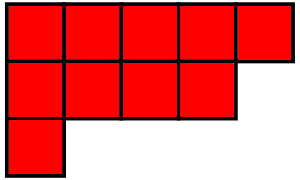### Seminar on This Week's FindsI wrote 300 issues of a colum called This Week's Finds, where I explained math and physics. This fall I'm running a seminar on selected topics from this column. For information on how to attend, go here.

Here you'll find lecture notes and videos. I'll keep updating and improving these notes.

#### Young diagrams and classical groups

Young diagrams are combinatorial structures that show up in a myriad of applications. Among other things, they can be used to classify conjugacy classes in the symmetric groups $$S_n$$, irreducible representations of $$S_n$$, and irreducible representations of the classical groups $$\mathrm{GL}(n)$$, $$\mathrm{SL}(n)$$, $$\mathrm{U}(n)$$ and $$\mathrm{SU}(n)$$.

#### Dynkin diagrams

Coxeter and Dynkin diagrams classify a wide variety of structures, most notably Coxeter groups, lattices having such groups as symmetries, and simple Lie algebras. The simply laced Dynkin diagrams also classify the Platonic solids and quivers with finitely many indecomposable representations. This tour of Coxeter and Dynkin diagrams will focus on the connections between these structures.

#### q-mathematics

A surprisingly large portion of mathematics generalizes to something called $$q$$-mathematics, involving a parameter $$q$$. For example, there is a subject called $$q$$-calculus that reduces to ordinary calculus at $$q = 1$$. There are important applications of $$q$$-mathematics to the theory of quantum groups and also to algebraic geometry over $$\mathbb{F}_q$$, the finite field with $$q$$ elements. These seminars will give an overview of $$q$$-mathematics and its applications.

#### The three-strand braid group

The three-strand braid group has striking connections to the trefoil knot, rational tangles, the modular group $$\mathrm{PSL}(2, \mathbb{Z})$$, and modular forms. This group is also the simplest of the Artin–Brieskorn groups, a class of groups which map surjectively to the Coxeter groups. The three-strand braid group will be used as the starting point for a tour of these topics.

#### Clifford algebras and Bott periodicity

The Clifford algebra $$\mathrm{Cl}_n$$ is the associative real algebra freely generated by $$n$$ anticommuting elements that square to -1. I will explain their role in geometry and superstring theory, and the origin of Bott periodicity in topology in facts about Clifford algebras.

#### The threefold and tenfold way

Irreducible real group representations come in three kinds, a fact arising from the three associative normed real division algebras: the real numbers, complex numbers and quaternions. Dyson called this the threefold way. When we generalize to superalgebras this becomes part of a larger classification, the tenfold way. We will examine these topics and their applications to representation theory, geometry and physics.

#### Exceptional algebras

Besides the three associative normed division algebras over the real numbers, there is a fourth one that is nonassociative: the octonions. They arise naturally from the fact that Spin(8) has three irreducible 8-dimensional representations. We will explain the octonions and sketch how the exceptional Lie algebras and the exceptional Jordan algebra can be constructed using octonions.

© 2022 John Baez
baez@math.removethis.ucr.andthis.edu